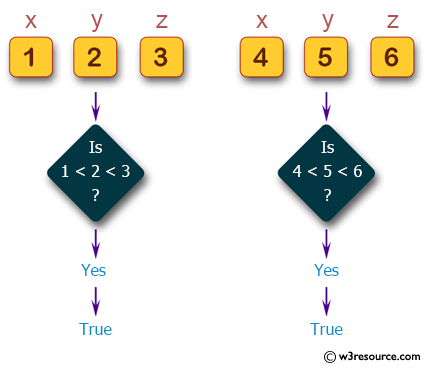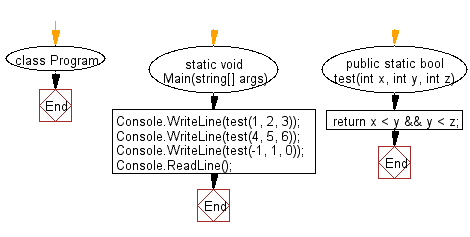﻿ C# - Check if y > x, z > y from three integers x, y, z# C# Sharp Basic Algorithm Exercises: Check whether y is greater than x, and z is greater than y from three given integers x,y,z

## C# Sharp Basic Algorithm: Exercise-48 with Solution

Write a C# Sharp program to check whether y is greater than x, and z is greater than y from three given integers x,y,z.

Pictorial Presentation:Sample Solution:-

C# Sharp Code:

``````using System;
using System.Linq;
namespace exercises
{
class Program
{
static void Main(string[] args)
{
Console.WriteLine(test(1, 2, 3));
Console.WriteLine(test(4, 5, 6));
Console.WriteLine(test(-1, 1, 0));
}
public static bool test(int x, int y, int z)
{
return x < y && y < z;
}
}
}
```
```

Sample Output:

```True
True
False```

Flowchart:C# Sharp Code Editor:

Improve this sample solution and post your code through Disqus

What is the difficulty level of this exercise?

Test your Programming skills with w3resource's quiz.

﻿